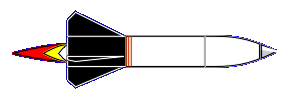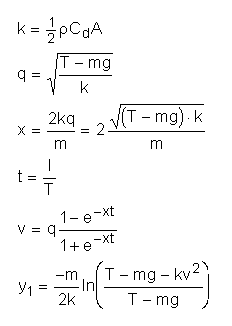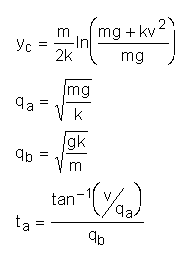# Rocket Equations Quick ReferenceEquations for finding your rocket's peak altitude and motor delay.Definition of Terms

• m = rocket mass in kg (see below)
• g = acceleration of gravity = 9.81 m/s2
• A = rocket cross-sectional area in m2
• Cd = drag coefficient = 0.75 for average rocket
• r (rho) = air density = 1.22 kg/m3
• t = motor burn time in seconds (NOTE: little t)
• T = motor thrust in Newtons (NOTE: big T)
• I = motor impulse in Newton-seconds
• v = burnout velocity in m/s
• y1 = altitude at burnout
• yc = coasting distance
• Note that the peak altitude is y1 + yc
• ta = coasting time => delay time for motorNote on the rocket mass: you usually know the empty (no motor) mass of your rocket mr. You can usually find the loaded mass of your motor, me, and the mass of the propellant, mp. Both Estes and Aerotech provide these numbers in their spec sheets and with the motors. Then

• average mass during boost is mr + me - mp/2
use this value for all but the yc, qa, and qb calculations.

• mass during coast is mr + me - mp
use this value for the yc, qa, and qb calculations.Back to Rocket Equations

## Questions

Your questions and comments regarding this page are welcome. You can e-mail Randy Culp (Tripoli #6926) for inquiries, suggestions, new ideas or just to chat.
Updated 24 August 2008